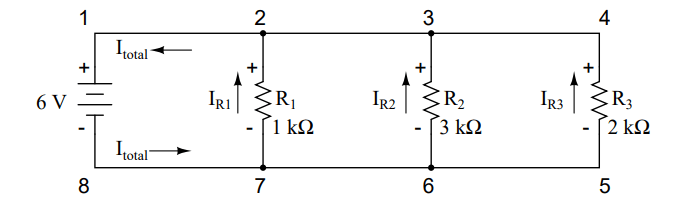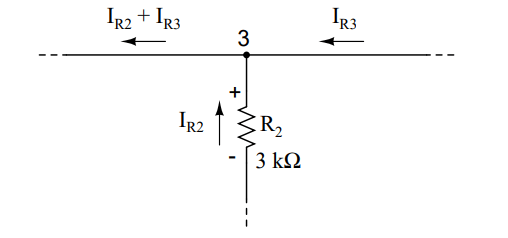# Kirchhoff’s Current Law (KCL)

### Kirchhoff’s Current Law Example

Let’s take a closer look at that last parallel example circuit:Solving for all values of voltage and current in this circuit:At this point, we know the value of each branch current and of the total current in the circuit. We know that the total current in a parallel circuit must equal the sum of the branch currents, but there’s more going on in this circuit than just that.

Taking a look at the currents at each wire junction point (node) in the circuit, we should be able to see something else:At each node on the negative ”rail” (wire 8-7-6-5) we have current splitting off the main flow to each successive branch resistor. At each node on the positive ”rail” (wire 1-2-3-4) we have current merging together to form the main flow from each successive branch resistor.

This fact should be fairly obvious if you think of the water pipe circuit analogy with every branch node acting as a ”tee” fitting, the water flow splitting or merging with the main piping as it travels from the output of the water pump toward the return reservoir or sump.

If we were to take a closer look at one particular ”tee” node, such as node 3, we see that the current entering the node is equal in magnitude to the current exiting the node:From the right and from the bottom, we have two currents entering the wire connection labeled as node 3. To the left, we have a single current exiting the node equal in magnitude to the sum of the two currents entering.

To refer to the plumbing analogy: so long as there are no leaks in the piping, what flow enters the fitting must also exit the fitting. This holds true for any node (”fitting”), no matter how many flows are entering or exiting.

### Formula

Mathematically, we can express this general relationship as such:

Iexiting = Ientering

Mr. Kirchhoff decided to express it in a slightly different form (though mathematically equivalent), calling it Kirchhoff’s Current Law (KCL):

Ientering + (-Iexiting) = 0

### Definition

Summarized in a phrase, Kirchhoff’s Current Law reads as such:

”The algebraic sum of all currents entering and exiting a node must equal zero”

That is, if we assign a mathematical sign (polarity) to each current, denoting whether they enter (+) or exit (-) a node, we can add them together to arrive at a total of zero, guaranteed.

Taking our example node (number 3), we can determine the magnitude of the current exiting from the left by setting up a KCL equation with that current as the unknown value:

I2 + I3 + I = 0

2 mA + 3 mA + I = 0

. . . solving for I . . .

I = -2 mA – 3 mA

I = -5 mA

The negative (-) sign on the value of 5 milliamps tells us that the current is exiting the node, as opposed to the 2 milliamp and 3 milliamp currents, which must both be positive (and therefore entering the node). Whether negative or positive denotes current entering or exiting is entirely arbitrary, so long as they are opposite signs for opposite directions and we stay consistent in our notation, KCL will work.

Together, Kirchhoff’s Voltage and Current Laws are a formidable pair of tools useful in analyzing electric circuits. Their usefulness will become all the more apparent in a later chapter (”Network Analysis”), but suffice it to say that these Laws deserve to be memorized by the electronics student every bit as much as Ohm’s Law.

#### Review:

• Kirchhoff’s Current Law (KCL): ”The algebraic sum of all currents entering and exiting a node must equal zero”